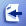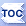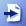# Math Parser for Objective CbcParserObjC is a math parser library written in Objective C. It can be used to build applications for iPhone (iOS) and OSX using XCode.

bcParserObjC can parse and evaluate mathematical formulas specified as strings at runtime. It supports variables and functions as well as literals and basic math operators. You can define your own custom variables and define your own functions. Variables can be defined before parsing, or they can be auto discovered in the expression and a callback you provide will decide the value of the newly discovered variable, or throw error if needed.

The help reference for bcParserObjC is here .

Here is an example of Objective C command line program using the bcParserObjC math parser library:

 ```#import #import "MathParser.h" // Resolves unknown variables: @interface MyVariableDelegate : NSObject @end @implementation MyVariableDelegate -(double) getVariableValue:(NSString *)varName {     if ([varName isEqualToString:@"A"]) {         return 5.0;     }     @throw [[NSException alloc] initWithName:@"UnknownVariableException"         reason:[NSString stringWithFormat:@"Variable '%@' is not defined.", varName] userInfo:nil]; } @end int main(int argc, const char * argv[]) {     @autoreleasepool {         NSLog(@"Testing MathParser");         MathParser *parser;         parser = [[MathParser alloc] init];         parser.expression = @"x+y/5";         [parser setVariable:@"X" withNewValue:5];         [parser setVariable:@"Y" withNewValue:10];         NSString *output;         output = [NSString stringWithFormat:@"%f", [parser evaluate]];         NSLog(@"%@", output);         parser.expression = @"x-sin(y)";         output = [NSString stringWithFormat:@"%f", [parser evaluate]];         NSLog(@"%@", output);         parser.expression = @" x * sum( 1,2, 3) ";         output = [NSString stringWithFormat:@"%f", [parser evaluate]];         NSLog(@"%@", output);         parser.optimizationOn = YES;         output = [NSString stringWithFormat:@"%f", [parser evaluate]];         NSLog(@"%@", output);         parser.expression = @" x * max( 1,2, y,3 ) ";         output = [NSString stringWithFormat:@"%f", [parser evaluate]];         NSLog(@"%@", output);         parser.expression = @"-x";         output = [NSString stringWithFormat:@"%f", [parser evaluate]];         NSLog(@"%@", output);         parser.expression = @"+y";         output = [NSString stringWithFormat:@"%f", [parser evaluate]];         NSLog(@"%@", output);         parser.expression = @"-3";         output = [NSString stringWithFormat:@"%f", [parser evaluate]];         NSLog(@"%@", output);         parser.expression = @"+4";         output = [NSString stringWithFormat:@"%f", [parser evaluate]];         NSLog(@"%@", output);         @try {             parser.expression = @"x+";             output = [NSString stringWithFormat:@"%f", [parser evaluate]];             NSLog(@"%@", output);         }         @catch (NSException *e) {             NSLog(@"Expected: %@", [e reason]);         }         parser.expression = @" (x / 3.4e-02) + y+x";         output = [NSString stringWithFormat:@"%f", [parser evaluate]];         NSLog(@"%@", output);         NSArray *vars = [parser getVariablesUsed];         for (NSString * varName in vars) {             NSLog(@"   Variable: %@", varName);         }         NSLog(@" Functions:");         NSArray *funcs = [parser getFunctions];         for (NSString * funcName in funcs) {             NSLog(@"  %@", funcName);         }         NSLog(@" Variables:");         vars = [parser getVariables];         for (NSString * varName in vars) {             NSLog(@"   defined: %@", varName);         }         parser.variableDelegate = [[MyVariableDelegate alloc] init];         // Using variable A whose value will be returned by variable delegate:         parser.expression = @"X+10/A";         output = [NSString stringWithFormat:@"%f", [parser evaluate]];         NSLog(@"%@", output);                 parser.expression = @"X+SIN(Y)/COS(X)";         [parser parse]; // parse before the loop.         NSDate *date = [NSDate date];         for (int i = 0; i< 1000000; i++) {             [parser setVariable:@"X" withNewValue:5+i];             [parser setVariable:@"Y" withNewValue:10+i];             [parser evaluate];         }         double timePassed_ms = [date timeIntervalSinceNow] * -1000.0;         NSLog(@"Milliseconds for 1 million evaluations: %f", timePassed_ms);     }     return 0; }  ```

## Math Parser Features

• Easy to use, simple Objective C class API.
• Function protocol to implement user defined functions.
• VariableDelegate protocol to provide values for undefined variables.
• Function/variable names start with letters and can contain letters, numbers and underscore (_).
• Functions with 0 or more known number of parameters in the form of: f(x,y,z)
• Functions with unknown number of parameters, e.g. SUM(a,b,c,)
• Comes with predefined math functions.
• Arithmetic Operators: +, -, /, *, ^(power), %(mod)
• Boolean Operators: <, >, =, &, |, ! ,<>, >=, <=
• Optimization: Constant expression elimination for repeated tasks
• Paranthesis: ( )
• Provides localization support which is a NSDictionary of message-keys to messages you can set.
• Optional locale specific decimal separator support (dot or comma).
• Comes as source code.
• Royalty free distribution.

Licensing is per developer. Once you purchase the bcParserObjC math parser library for Objective C, you can ship the binaries royalty free with your applications. The source code is provided so that you can change to fit your needs. bcParserObjC license can be viewed here .

## Purchasing bcParserObjC Math Parser Library

You can pay with credit card and download bcParserObjC immediately from our online store for only \$29.95. Full version includes Objective C source code. Upgrades are free for registered users. Licensing is per developer. Site license option is available in the online store page. You can deploy the the component royalty free with your applications as many times as you want. Site license allows any number of developers use the component at your development site. Site License is \$290.95 Site licenses can be purchased here.